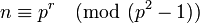# Number of conjugacy classes in group of prime power order is congruent to order of group modulo prime-square minus one

(diff) ← Older revision | Latest revision (diff) | Newer revision → (diff)

## Statement

Suppose$p$ is a prime number and$P$ is a group of prime power order with underlying prime$p$. Then the number of conjugacy classes of$P$ (which is the same as the number of irreducible representations) is congruent to the order of$P$ modulo$(p^2 - 1)$.

## Facts used

1. Number of irreducible representations equals number of conjugacy classes
2. Degree of irreducible representation divides order of group: For a$p$-group, in particular, this means that it is a power of$p$.
3. Sum of squares of degrees of irreducible representations equals order of group

## Proof

Given: A prime number$p$. A finite$p$-group$P$ of order$p^r$ with$n$ conjugacy classes.

To prove:$n \equiv p^r \pmod{(p^2 - 1)}$

Proof:

Step no. Assertion/construction Facts used Given data used Previous steps used Explanation
1$n$ equals the number of irreducible representations of$P$. Fact (1)$n$ is the number of conjugacy classes.
2 All irreducible representations of$P$ have degree$p^i$ for some nonnegative integer$i$, with$0 \le i \le r$. Fact (2)$P$ has order$p^r$ [SHOW MORE]
3 Let$a_i$ be the number of irreducible representations of degree$p^i$ for$i = 0,1,2,\dots,r$. Then,$a_0 + a_1 + a_2 + \dots + a_r = n$. Steps (1), (2) Step-combination direct.
4$a_0(p^0)^2 + a_1(p^1)^2 + a_2(p^2)^2 + \dots + a_r(p^r)^2 = p^r$ Fact (3)$P$ has order$p^r$ Step (3) Step-fact direct.
5$a_i \equiv a_i(p^i)^2 \pmod{(p^2 - 1)}$ [SHOW MORE]
6$a_0 + a_1 + a_2 + \dots + a_r \equiv a_0(p^0)^2 + a_1(p^1)^2 + a_2(p^2)^2 + \dots + a_r(p^r)^2 \pmod{(p^2 - 1)}$ Step (5) Sum up Step (5) for$0 \le i \le r$.
7$n \equiv p^r \pmod{(p^2 - 1)}$ Steps (3), (4), (6) Step-combination direct.